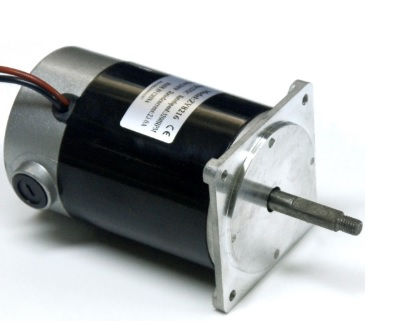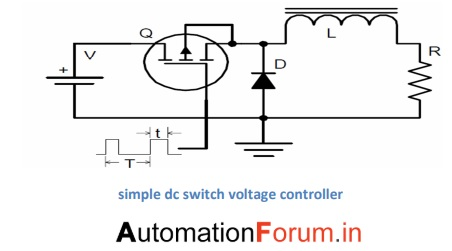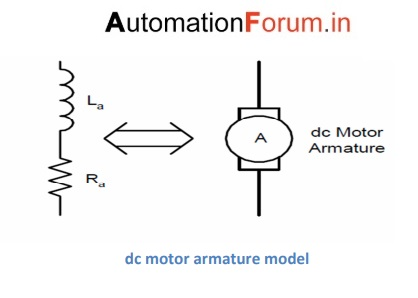# How to control speed of dc motor

## DC Motor Workng Principle

A machine that converts DC power into mechanical energy is known as a DC motor. Its operation is based on the principle that when a current-carrying conductor is placed in a magnetic field, the conductor experiences a mechanical force. The direction of the force is given by the rule of Fleming’s left hand.# Speed control of dc motor

The speed control of a motor means the intentional variation of speed according to the requirement of the work load connected to the motor. This can be done by mechanical means, such as the use of stepped pulleys, a set of shift gears, a friction clutch mechanism, etc. However, speed control by electrical means has greater advantages than mechanical speed controls. DC motors offer easy speed control and that is why DC motors are preferable to other types of motors in many applications. Several methods of speed control can be obtained from its expression which is:

N=V - IaRa/Kϕ

Where:-

N=speed of motor Ia=armature current Ra=armature resistance Φ=field flux

So it can be concluded that speed of dc motor depends upon

a. The applied voltage b. The field flux c. Drop in armature circuit resistance IaRa

And accordingly speed can be controlled by varying the above factors.

## A. Speed control by varying the field flux:-

In shunt field winding motor ,variable resistor called field regulator is connected in series while in series motor a resistor called diverter is connected in parallel with series field winding.When the resistance of the field circuit is varied, the field current and the flux vary. But when introducing the field regulator, the resistance of the field circuit can only be increased, that is, the field flow can only be decreased and, therefore, the engine speed can be increased. It is not possible to decrease the speed of the motor with this method. Similarly, by increasing the resistance of the diverter circuit, the field current can be reduced and the speed of the motor cabin in series can be increased. Speed reduction is not possible using the diverter.

## B. Speed control by connecting a resistance in series with armature:-

A resistor called a controller is connected in series with the armature. Here the motor speed can be reduced as desired. By using the desired value of the controller resistance, the speed can be reduced or greatly increased. The field winding must be connected through the supply terminals, otherwise the flow produced will be severely affected and it may not be enough torque to rotate the motor. The disadvantages of this method are as follows:-

a. The overall efficiency of the system is low as much of the input energy is dissipated in the controller as heat. b. The controller has relatively high cost. c. The speed may vary largely with variation of load.

## C. Speed control by controlling the voltage applied across the armature terminals:-

In this method of speed control the armature is supplied with a variable voltage with the help of a motor-generator set since the supply voltage available from the electricity authority cannot be varied at will.This system of speed control is also known as the Ward-Leonard system.If a reversing switch is incorporated,by changing the polarity of the armature supply terminals,speed can be varied in the opposite direction also.This system is advantageous over other system in following ways:-

a. it provides smooth control of speed over a wide range in both directions b. The system is more efficient at low speeds as there are no resistors connected in series with the armature circuit.

We apply this strategy only to control the motor speed as other methods are lossy and inefficient. Here instead of adding extra motor- generator set we control speed by PLC. The PLC supplies control signals to the MOSFET switches .These control signals have variable duty cycle that depends on the speed required. Depending on the duty cycle the motor gets the average voltage and accordingly speed varies.

Consider the circuit shown below .Here we have a dc voltage source V, a resistor R, inductor L, diode D, and a semiconductor switch Q (shown here as an N-channel insulated gate MOSFET). The signal applied to the gate of the switch Q is a pulse train with constant frequency f (and constant period T), but with varying pulse width t. The amplitude of the signal applied to the gate will cause the switch to transition between cutoff and saturation with very short rise and fall times. The relative values of R and L are selected such that the time constant = L/R is at least 10 times the period T of the pulse train applied to the gate of Q. The long L/R time constant will have a low-pass filtering effect on the chopped output of the switch Q, and will effectively smooth the current into dc with very little ac component.For switch Q, the relationship between the time of connection t and the period T is defined as the duty cycle, and is represented as a percentage between zero and 100%. For any work cycle between 0% and 100%, the average voltage of the resistance will be a corresponding percentage of the voltage V and also the power transferred to the motor. For example, if we adjust the door pulses applied so that The duty cycle is 35% (ie, on 35% of the time, off 65% of the time), then the voltage in the resistance R will be 35% of the input voltage V.

This is because, during the time that the switch is ON, the inductor L will store energy; during the time the switch is OFF, the inductor it will give up part of its stored energy keeping the current flowing in the circuit through the inductor L, resistance R, and the forward polarized diode D (in this application, the diode is called freewheeling) diode or switching diode). As in the figure below we have resistance and inductor connected in series we can model dc armature as concentrated resistance and inductance concentrated in seriesSince we can model the dc motor armature as a series resistance and inductance, we can substitute the armature in place of the resistor and inductor in our dc switch circuit which looks as

## Ladder diagram

For generation of pulses ,first whole time period is set in counter by any n-bit according to resolution required. Now according to the percentage of rpm required with respected to maximum rpm of the motor , the counter is also set at the same percentage(value with respect to n-digit maximum value) as the voltage. We have comparator to check that value. Till fixed value signals passes while it remains off for remaining period of the pulse. In this way we generate signals of varying duty cycle pulses.

The ladder diagram when simulated gave the same result .Signal remained ON until the counter finished counting and became off for rest period.The process is repeated in every time period.

## Matlab Simulink :-

We used matlab Simulink to study how the rpm of motor varied by changing the dc average supply to the motor and this dc supply voltage was varied by supplying the variable duty cycle signal through PLC